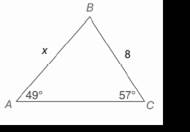Chapter 11.CR, Problem 9CRElementary Geometry For College St...

7th Edition
Alexander + 2 others
ISBN: 9781337614085

Solutions

Chapter
SectionElementary Geometry For College St...

7th Edition
Alexander + 2 others
ISBN: 9781337614085
Textbook Problem

In Review Exercises 9 to 12, use the Law of Sines or the Law of cosines to find the indicated length of side or angle measure. Angle measures should be found to the nearest degree; distances should be found to the nearest tenth of a unit.To determine

To find:

The indicated length of side or angle measure using the law of sines or the law of cosines.

Explanation

Procedure used:

In any triangle ABC such that

AB=c,

BC=a,

CA=b,

mA=α,

mB=β, and

mC=γ.

The Law of cosines is given by

a2=b2+c2-2bccosα

b2=c2+a2-2cacosβ

c2=a2+b2-2abcosγ

The Law of sines is given by

asinα=bsinβ=csinγ

Calculation:

Given:

Still sussing out bartleby?

Check out a sample textbook solution.

See a sample solution

The Solution to Your Study Problems

Bartleby provides explanations to thousands of textbook problems written by our experts, many with advanced degrees!

Get Started

For the following set of scores, find the value of each expression: a. X b. (X)2 c. X2 d. (X +3)

Essentials of Statistics for The Behavioral Sciences (MindTap Course List)

Solve the equations in Exercises 126. (x3+1)x+1(x3+1)2x+1=0

Finite Mathematics and Applied Calculus (MindTap Course List)

If f(x)=x2xx1andg(x)=x is it true that f = g?

Single Variable Calculus: Early Transcendentals, Volume I